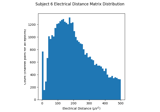# mne.preprocessing.compute_bridged_electrodes#

mne.preprocessing.compute_bridged_electrodes(inst, lm_cutoff=16, epoch_threshold=0.5, l_freq=0.5, h_freq=30, epoch_duration=2, bw_method=None, verbose=None)[source]#

Compute bridged EEG electrodes using the intrinsic Hjorth algorithm.

First, an electrical distance matrix is computed by taking the pairwise variance between electrodes. Local minimums in this matrix below lm_cutoff are indicative of bridging between a pair of electrodes. Pairs of electrodes are marked as bridged as long as their electrical distance is below lm_cutoff on more than the epoch_threshold proportion of epochs.

Based on  and the EEGLAB implementation.

Parameters:
instinstance of Raw, Epochs or Evoked

The data to compute electrode bridging on.

lm_cutofffloat

The distance in $${\mu}V^2$$ cutoff below which to search for a local minimum (lm) indicative of bridging. EEGLAB defaults to 5 $${\mu}V^2$$. MNE defaults to 16 $${\mu}V^2$$ to be conservative based on the distributions in Greischar et al..

epoch_thresholdfloat

The proportion of epochs with electrical distance less than lm_cutoff in order to consider the channel bridged. The default is 0.5.

l_freqfloat

The low cutoff frequency to use. Default is 0.5 Hz.

h_freqfloat

The high cutoff frequency to use. Default is 30 Hz.

epoch_durationfloat

The time in seconds to divide the raw into fixed-length epochs to check for consistent bridging. Only used if inst is mne.io.BaseRaw. The default is 2 seconds.

bw_methodNone

bw_method to pass to scipy.stats.gaussian_kde.

verbose

Control verbosity of the logging output. If None, use the default verbosity level. See the logging documentation and mne.verbose() for details. Should only be passed as a keyword argument.

Returns:
bridged_idx

The indices of channels marked as bridged with each bridged pair stored as a tuple.

ed_matrixndarray of float, shape (n_epochs, n_channels, n_channels)

The electrical distance matrix for each pair of EEG electrodes.

Notes

New in v1.1.

References

## Examples using mne.preprocessing.compute_bridged_electrodes#Identify EEG Electrodes Bridged by too much Gel

Identify EEG Electrodes Bridged by too much Gel